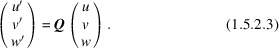International
Tables for
Crystallography
Volume A
Space-group symmetry
Edited by M. I. Aroyo

International Tables for Crystallography (2015). Vol. A, ch. 1.5, p. 83

## Section 1.5.2.1. Covariant and contravariant quantities

H. Wondratscheka and M. I. Aroyob

#### 1.5.2.1. Covariant and contravariant quantities

| top | pdf |

If the direct or crystal basis is transformed by the transformation matrix P: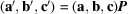, the corresponding basis vectors of the reciprocal (or dual) basis transform as (cf. Section 1.3.2.5)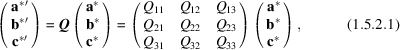where the notation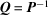is applied (cf. Section 1.5.1.2).

The quantities that transform in the same way as the basis vectors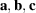are called covariant with respect to the basisand contravariant with respect to the reciprocal basis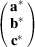. Such quantities are the Miller indices (hkl) of a plane (or a set of planes) in direct space and the vector coefficients (h, k, l) of the vector perpendicular to those planes, referred to the reciprocal basis: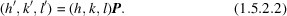Quantities like the vector coefficients of any vector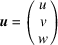in direct space (or the indices of a direction in direct space) are covariant with respect to the basis vectorsand contravariant with respect to: